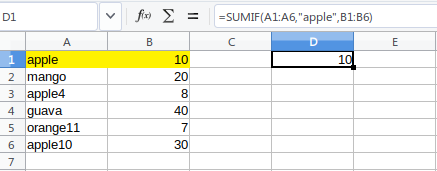# SUMIF and SUMIFS Function with Examples in LibreOffice Calc

This tutorial explains basics of SUMIF and SUMIFS functions in LibreOffice Calc.

## SUMIF

SUMIF Function returns the sum total of the values from a range/list of cells based on a condition. For example, if you have a list of numbers in Calc and wants to sum only the values which are less than 20, then you can use SUMIF function.

Here are some examples which helps you to understand the workings of SUMIF.

## SUMIF Examples

#### Using Numbers

The below example sums the values from cells A1 to A6 if it is less than 20. The yellow highlighted values are the cells which is matching with the conditions.

=SUMIF(A1:A6,”<20”)You can also keep the criteria value i.e. 20 in a cell and use the cell reference in the SUMIF formula as below. It gives same result as above.

=SUMIF(A1:A6,”<“&E1)SUMIF can take three arguments where based on the criteria match in a range, you can sum another range. Below example, searches 10 in A1 to A6 and if match is found it returns corresponding sums from range B1 to B6.

=SUMIF(A1:A6,10,B1:B6)Similarly you can put value 10 in any cell and use the cell reference in above formula.

#### Using Texts

You can also search texts as well similar way and return the sum.

The following example searches “apple” in the range A1:A6 and returns the sum of matching entries from corresponding sum range.

=SUMIF(A1:A6,”apple”,B1:B6)Using below condition, you can sum the values that are NOT equal to a specific text/string. The NOT operator is <>. Please note that <> operator is inside the text with “.

=SUMIF(A1:A6,”<>apple”,B1:B6)To search using a single wild card character, you can use ? in the condition. E.g. if you want to sum only strings matching with “apple4” and not “apple10” or “apple”, use below example.

=SUMIF(A1:A6,”apple?”,B1:B6)You can also use wild card * to search any number of characters in a range of cells and returns sum using SUMIF. Below example sums all matching cells where apple word is found.

=SUMIF(A1:A6,”*apple*”,B1:B6)## SUMIFS Example

You can use SUMIFS for multiple criteria ranges for summing up values. SUMIFS takes first argument as the range which to be summed and next the set of criteria as per above examples.

In below example, it returns the sum of revenue when sales are less than equal to 20 and product name start with pen.

=SUMIFS(C2:C6,A2:A6,”pen*”,B2:B6,”<=20″)Note:

• SUMIFS conditions are evaluated as AND and sum is returned only when all conditions are satisfied.
• SUMIFS conditions ranges should be of same length.
• You can specify at most 127 conditions pairs in SUMIFS.

## Summary

This guide definitely would help you understand the working pricciples of SUMIF and SUMIFS. You can experiment with more conditions as per your need in LibreOffice Calc. Drop a comment below if you have any questions.

#### Looking for something else?

Read our complete tutorial index of Calc, Writer, Impress and Draw.

Be an expert on LibreOffice.

Small and quality tutorials. Delivered. Thrice a week.

## 6 thoughts on “SUMIF and SUMIFS Function with Examples in LibreOffice Calc”

1.Brian Ferrand says:

I am not able to get sumif to work when comparing to cells with text/string contents. It works fine if I compare to “apple” but not if I compare to cell C5 which contains the word “apple”,. Am I overlooking something?

2.Carlo says:

The formulas on this guide are not working for me (Libreoffice Version: 6.0.7.3 Ubuntu), I had to replace the commas with semicolons.

1.Arindam Giri says:

It should work with both comma and semicolons.

2.bruno says:

maybe because of your language. in my case( brazil), we also need to change it, because we use comma to separate decimals

3.Ubirajara Bandeira Jr says:

It would be great if it functioned… 😦

I cannot use dates to compare, although internally they are only integer values (the value is always 0 when I try to use dates). I cannot use wildcards on text comparations (the result is always 0 when I try to use text with wildcards)…

I’m using 6.2.6.2 LO version.

“sumifs” is so buggy…

4.Mike says:

Very new to using a spreadsheet and have very limited knowledge how to resolve this. Attempted to solve this with IF and IFS and SUMIF which seemed like it might be the best way. If you could point me in the correct direction it would be appreciated.

D3 is “yes” or “no” (this is a manual entry, effectively true or false)

If “yes” F3 = C3/B3

If “no” G3 = G3+C3

This site uses Akismet to reduce spam. Learn how your comment data is processed.